[[d.project_title]][[d.material_name]]# #REMAKE再造 第二轮 SMARS小车巡迹游https://makelog.dfrobot.com.cn/article-309740.html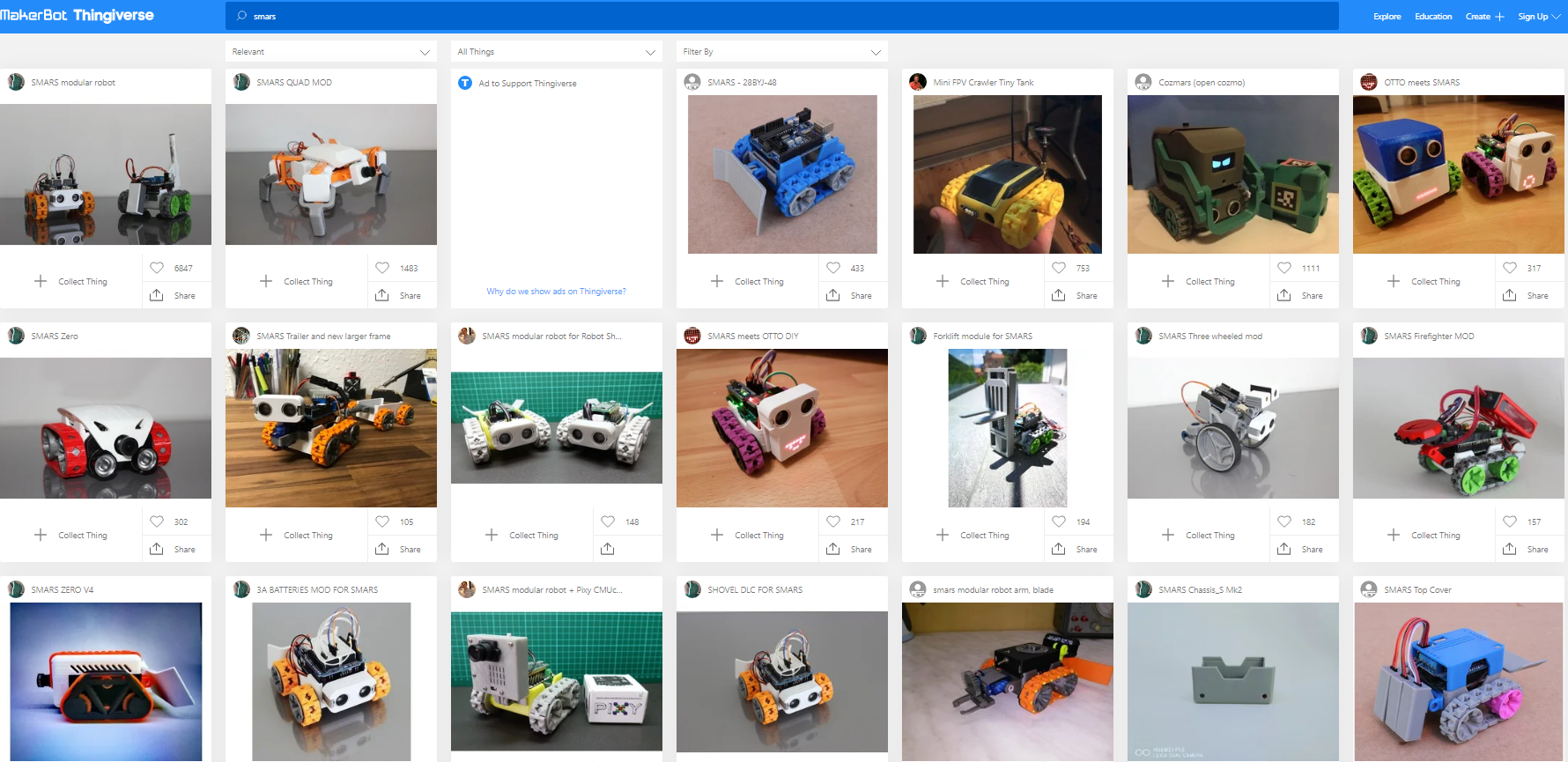SMARS作为一个全打印的小车，要搞巡迹是有缺点的。首先最大的缺点是它的履带很硬，摩擦系数小，与硬质地面接触面积还小，这样摩擦力过小，在纠正车体偏移过程中很容易打滑。其次，还是由于履带的原因，小车在行驶过程中，地面灰度传感器相对地面会有上下的起伏。而传感器返回数值和地面距离紧密关联，这都给稳定巡迹带来难度。还有小车空间紧张，供电电压偏低等等缺点，我们一 一克服。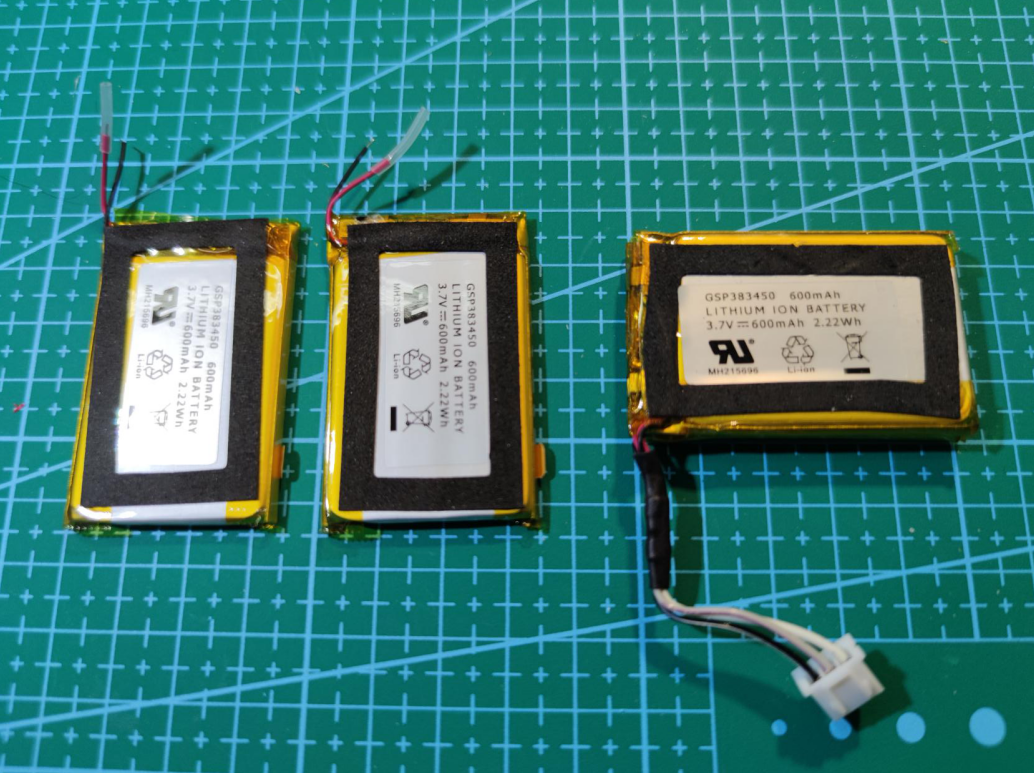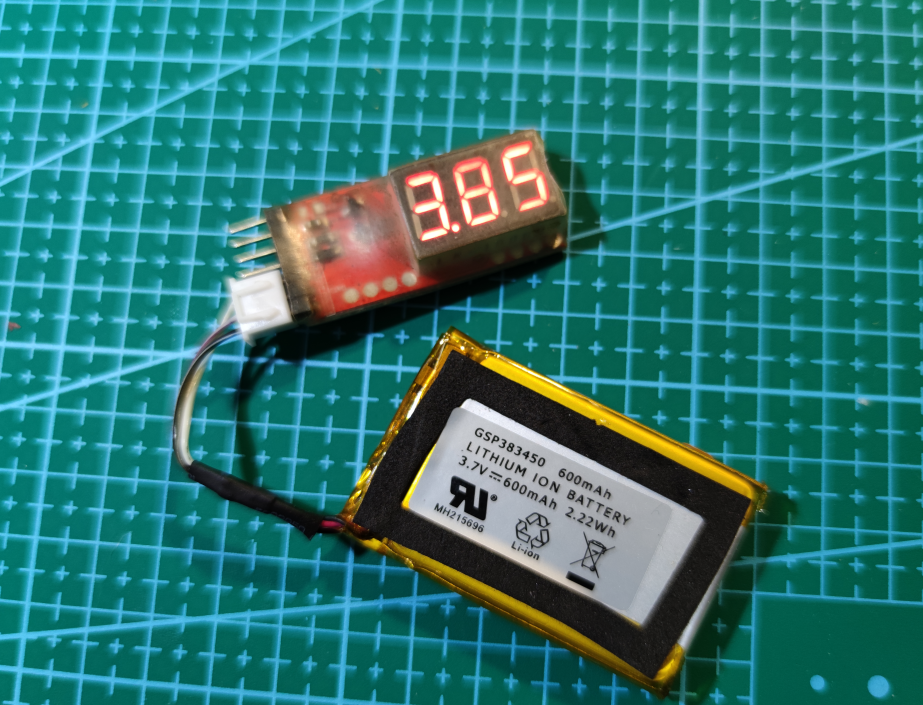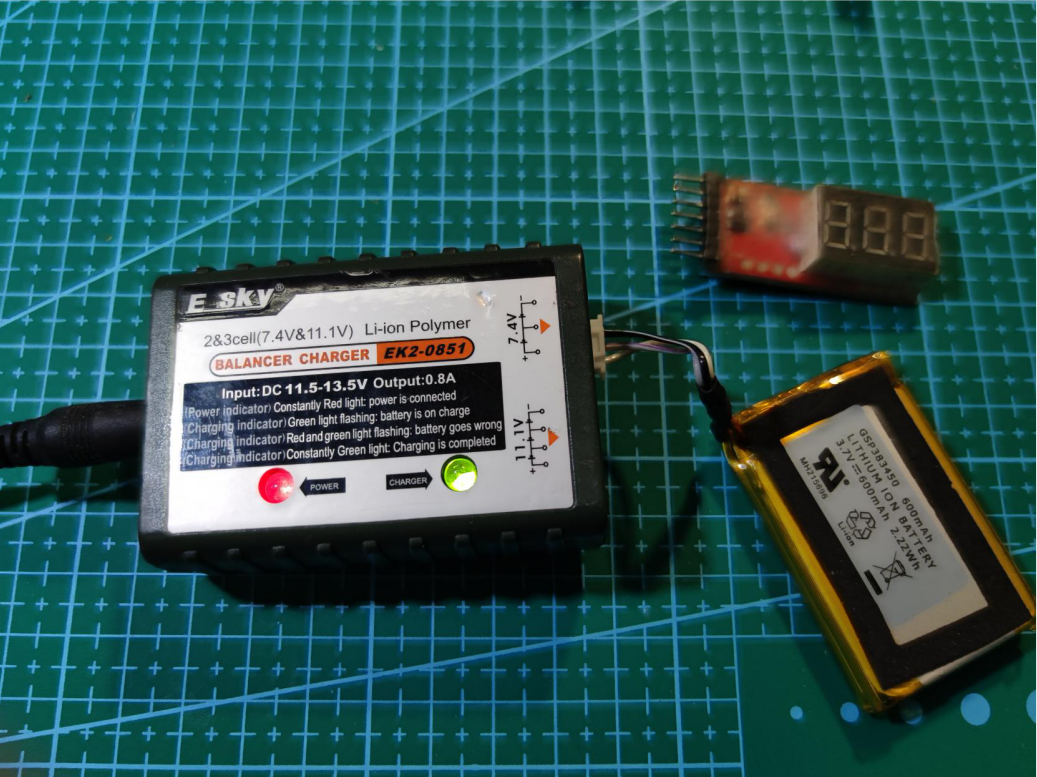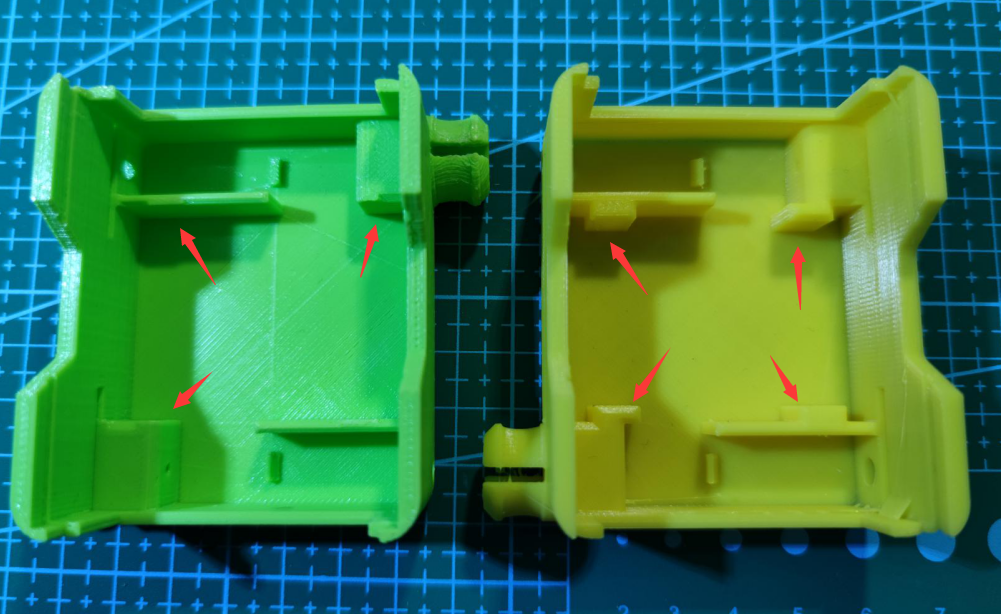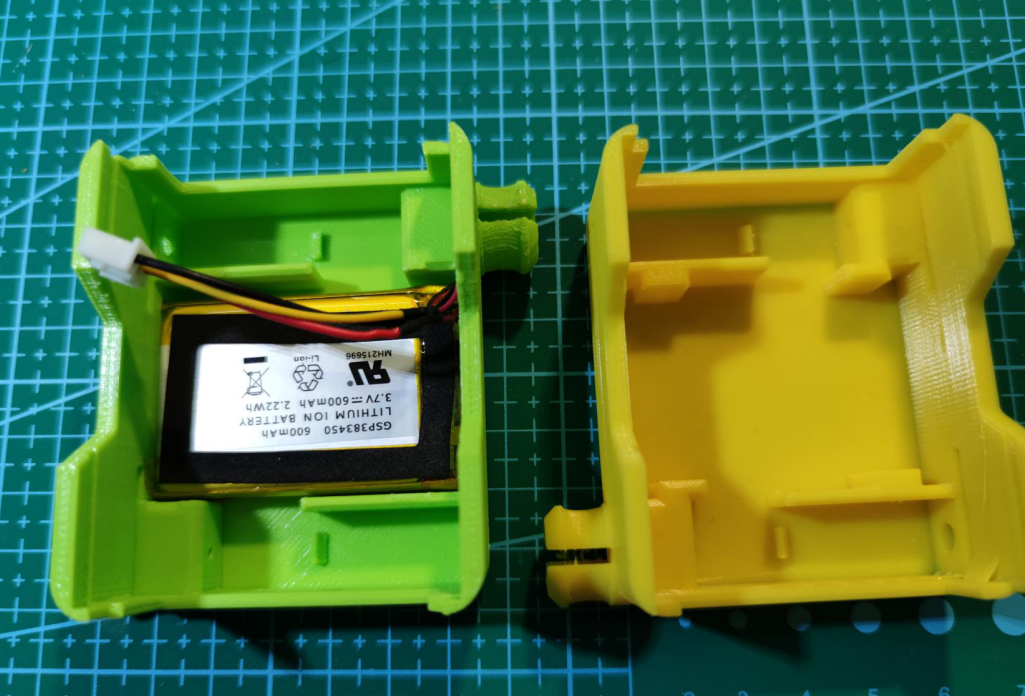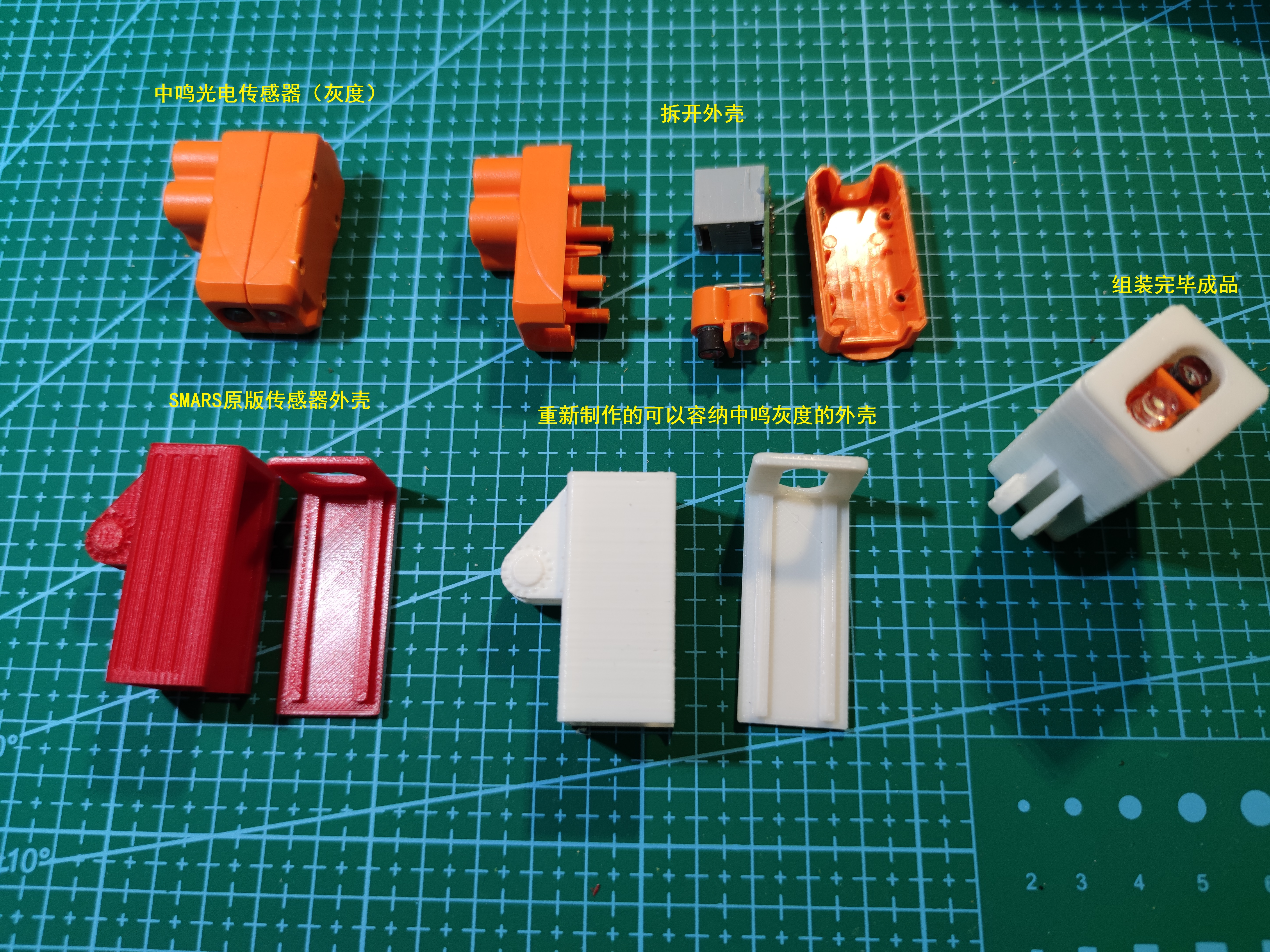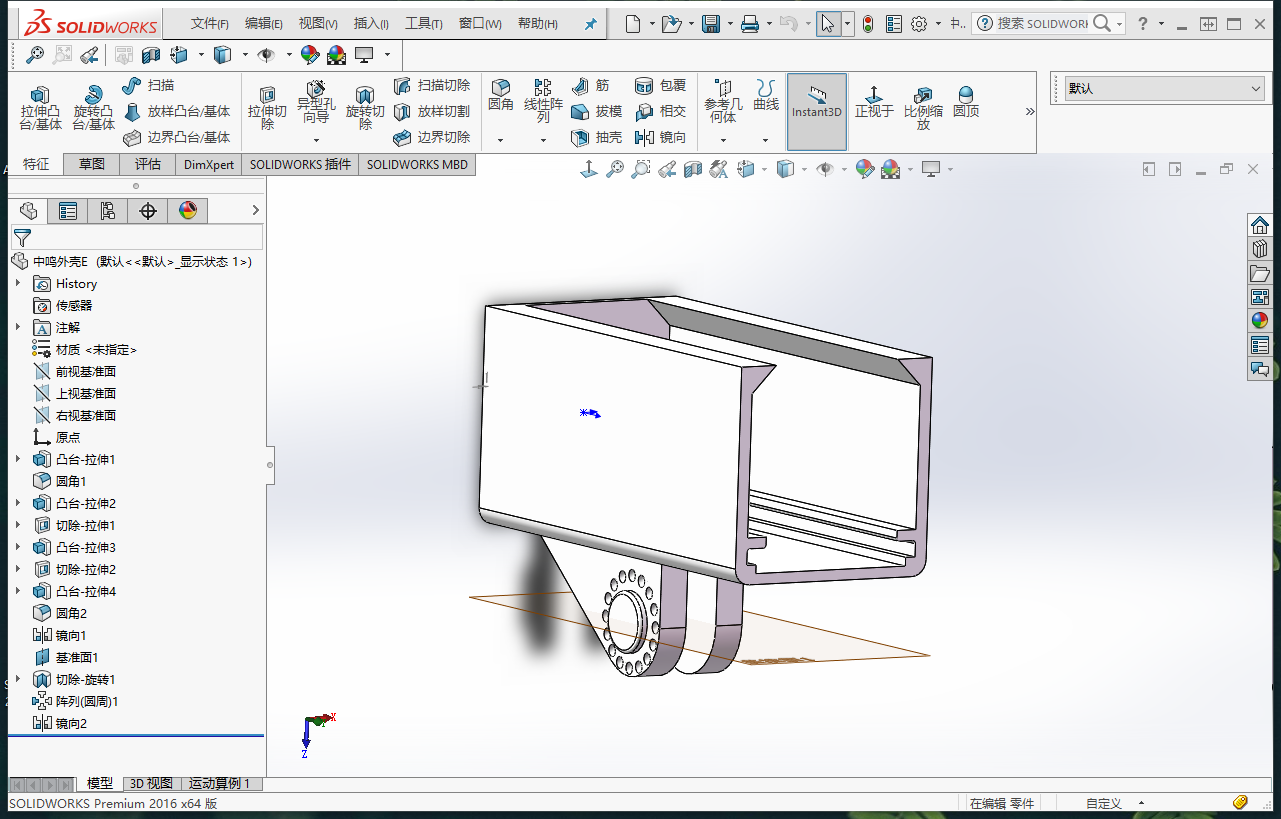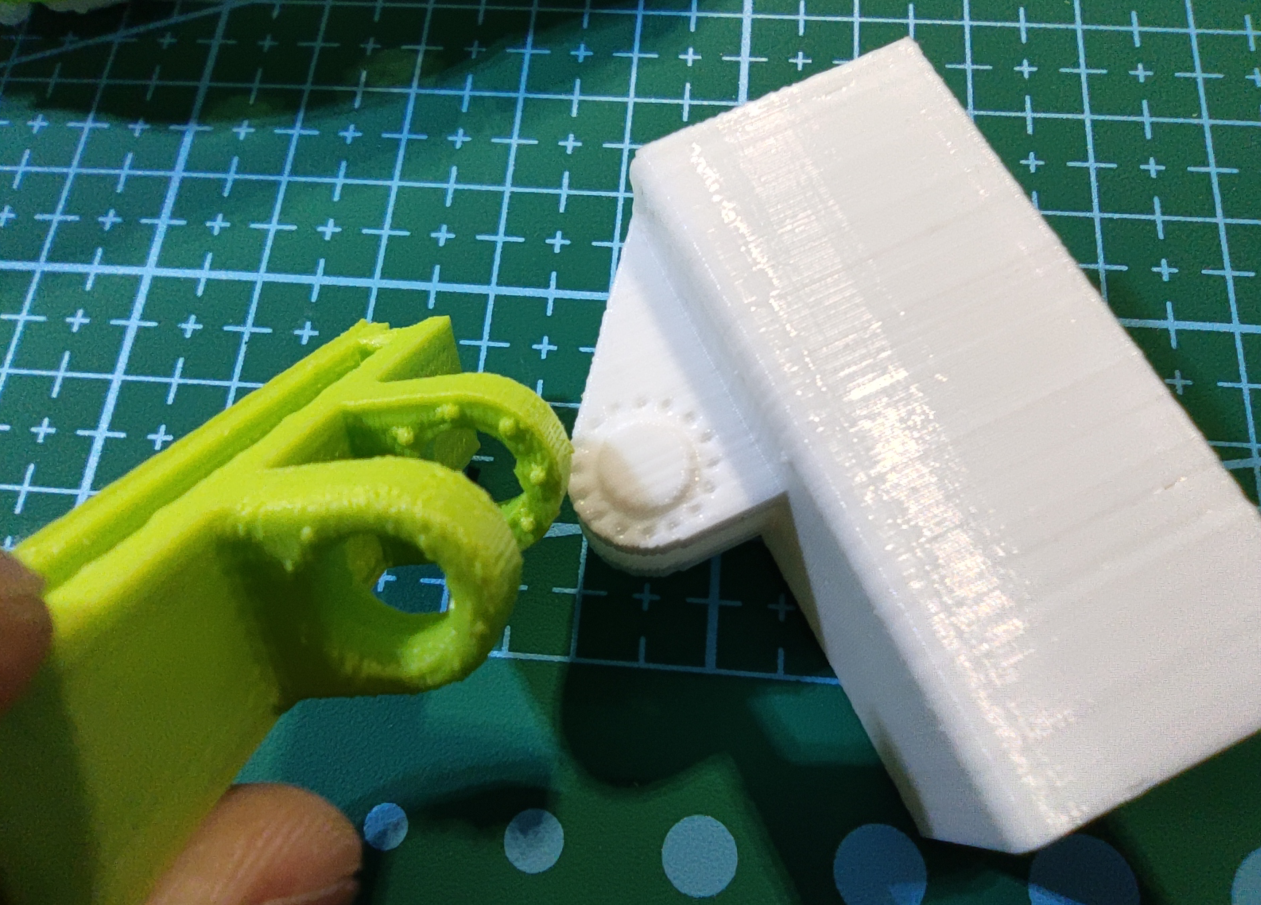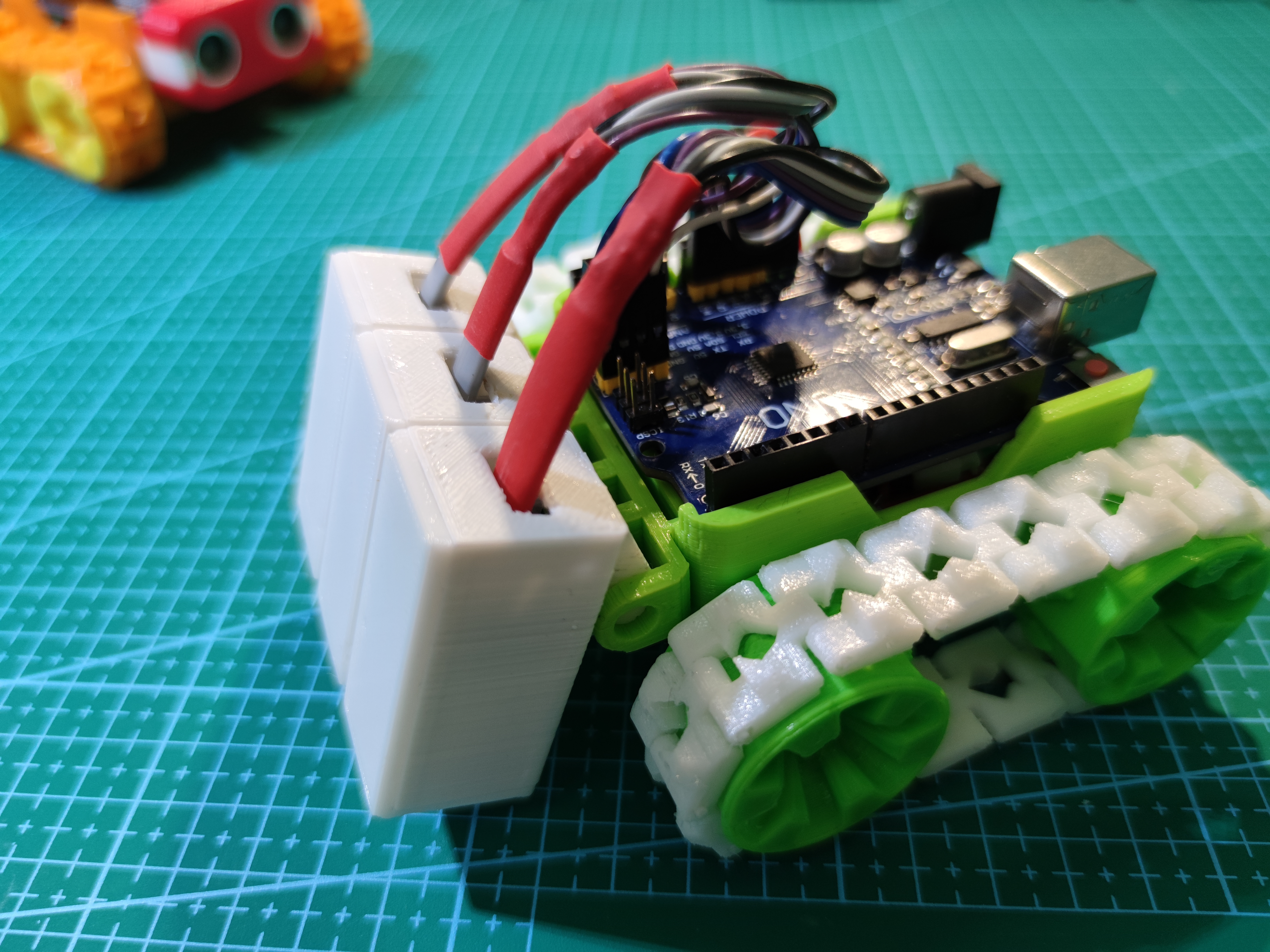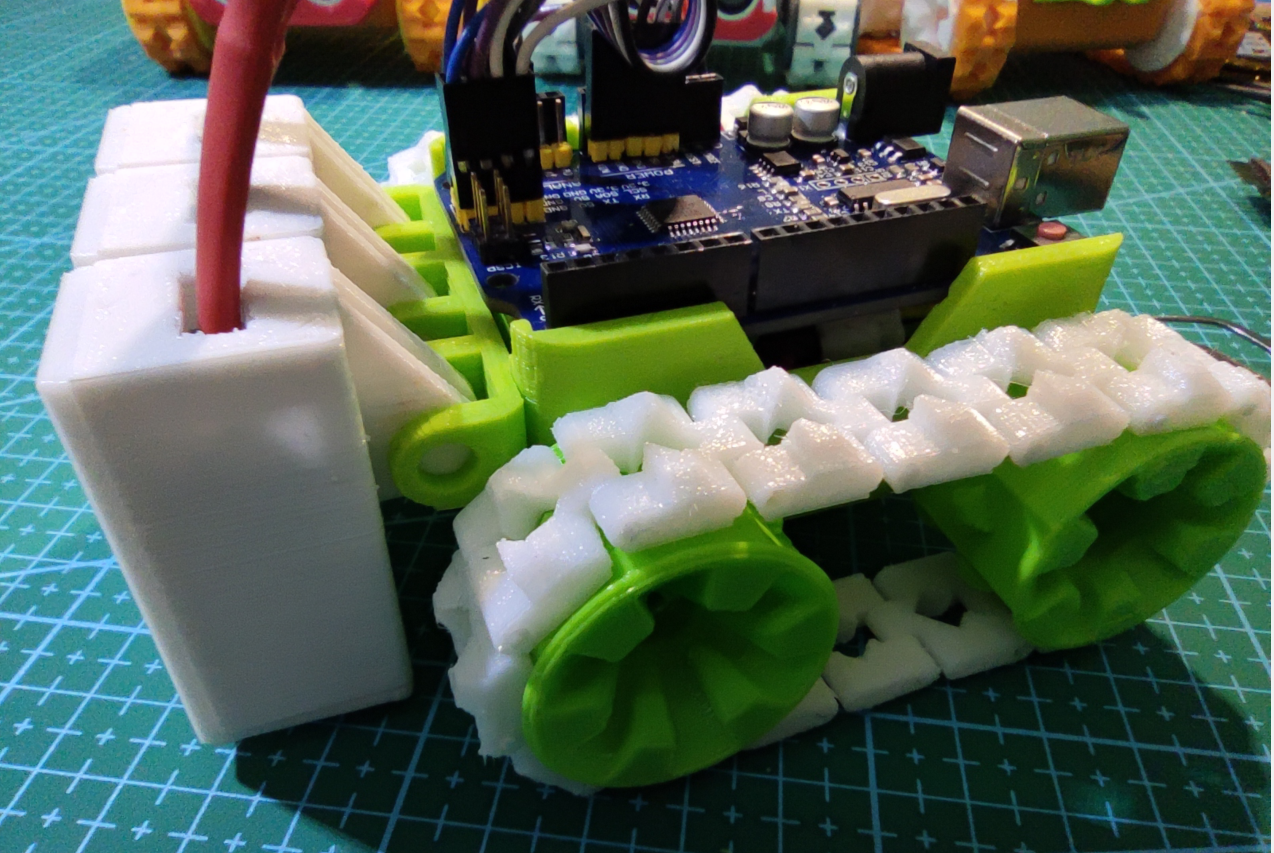```	                    					```/* Smars 小车
巡迹测试
阿风
2021.02.13C
*/
{
extern int P1,P2,P3;
extern int S1,S2,S3;

if(P1<300){    //和阈值比较，转化为数字量
S1=1;
}
else{
S1=0;
}
if(P2<300){
S2=1;
}
else{
S2=0;
}
if(P3<150){
S3=1;
}
else{
S3=0;
}
}

void go (int a,int b)//-255~255  (MX1508 0,0停止  1,1刹车）left 5~ 4  right 6~ 7 555刹车
{
if (a==0){
digitalWrite(5,0);
digitalWrite(4,0);
}
else{
if (a==555){          //刹车
digitalWrite(5,1);
digitalWrite(4,1);
}
else{
if (a>0){
analogWrite(5,a);
digitalWrite(4,0);
}
else{
analogWrite(5,a-255);
digitalWrite(4,1);
}
}
}
if (b==0){
digitalWrite(6,0);
digitalWrite(7,0);
}
else{
if (b==555){          //刹车
digitalWrite(6,1);
digitalWrite(7,1);
}
else{
if (b>0){
analogWrite(6,b);
digitalWrite(7,0);
}
else{
analogWrite(6,b-255);
digitalWrite(7,1);
}
}
}
}
int incomedate = 0; //串口数据变量
int P1,P2,P3; //左，中，右传感器模拟值
int S1,S2,S3; //左，中，右传感器数字值
int leftspeed,rightspeed; //左马达速度，右马达速度
int V=110; //基础巡线速度
int PID_P=8; //pid  p参数
int ZuoYou=1;  //0左 1右 黑线的左右
int slow=55;   //摇摆巡线慢速
int fast=130;   //摇摆巡线快速

void setup()
{
pinMode(13,OUTPUT);
pinMode(4, OUTPUT);
pinMode(5, OUTPUT);
pinMode(6, OUTPUT);
pinMode(7, OUTPUT);
pinMode(1, OUTPUT);
pinMode(2, OUTPUT);
Serial.begin(9600); //设置串口波特率9600
digitalWrite(13,0);

digitalWrite(1,0);  //P1 指示灯
digitalWrite(2,0);  //P3 指示灯
delay(3000);
}

void loop()
{
//digitalWrite(13,1);
while(1)
{
if (ZuoYou==1) //沿线右侧走
{
if (S3==1)
{
ZuoYou=0;
continue;
}
else
{
if (S2==1)  //黑线上
{
go(fast,slow+5);
}
else
{
go(slow,fast+5);
}
}
}
else
{
if (S1==1)
{
ZuoYou=1;
continue;
}
else
{
if (S2==1)  //黑线上
{
go(slow,fast+5);
}
else
{
go(fast,slow+5);
}
}
}
}
go(leftspeed,rightspeed);
}```
```

Makelog作者原创文章，未经授权禁止转载。
8
1[[c.user_name]] [[c.create_time]]
[[c.parent_comment.count]]
|
[[c.comment_content]]
[[r.user.user_name]] [[r.create_time]]
[[r.comment_content]]

2
16
5
7
0
10
1
6
2
5